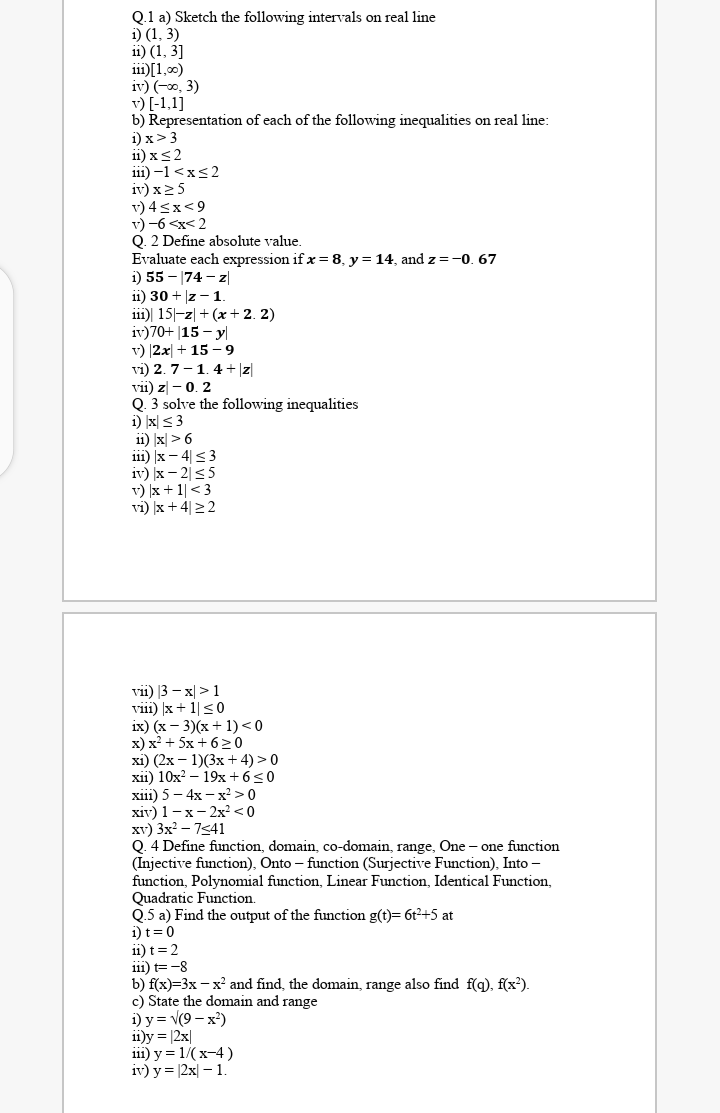# Q.1 a) Sketch the following intervals on real line i) (1, 3) 11) (1, 3] i11)[1,00) iv) (-0, 3) v) [-1,1] b) Representation of each of the following inequalities on real line: i) x>3 11) xs2 i11) –1 6 i11) |x – 4|<3 iv) x – 2|<5 v) |x + 1|<3 vi) x +4|22 vii) |3 – x| >1 viii) )x + 1|<0 ix) (х — 3)(х + 1) <0 x) x² + 5x + 6>0 xi) (2x – 1)(3x + 4) > 0 xii) 10x? – 19x + 6<0 xiї) 5 — 4х — х? > 0 хiv) 1 — х — 2х? <0 xv) 3x? – 7541 0. 4 Define function, domain, co-domain, range, One - one function (Injective function), Onto – function (Surjective Function), Into - function, Polynomial function, Linear Function, Identical Function, Quadratic Function. Q.5 a) Find the output of the function g(t)= 6t2+5 at i) t=0 ii) t=2 111) t= -8 b) f(x)=3x - x' and find, the domain, range also find f(g), f(x²). c) State the domain and range i) у%3D V(9 — х?) ii)y = |2x| iil) у %3D 1/(х-4) iv) y = |2x| – 1

Questionhelp_outlineImage TranscriptioncloseQ.1 a) Sketch the following intervals on real line i) (1, 3) 11) (1, 3] i11)[1,00) iv) (-0, 3) v) [-1,1] b) Representation of each of the following inequalities on real line: i) x>3 11) xs2 i11) –1 6 i11) |x – 4|<3 iv) x – 2|<5 v) |x + 1|<3 vi) x +4|22 vii) |3 – x| >1 viii) )x + 1|<0 ix) (х — 3)(х + 1) <0 x) x² + 5x + 6>0 xi) (2x – 1)(3x + 4) > 0 xii) 10x? – 19x + 6<0 xiї) 5 — 4х — х? > 0 хiv) 1 — х — 2х? <0 xv) 3x? – 7541 0. 4 Define function, domain, co-domain, range, One - one function (Injective function), Onto – function (Surjective Function), Into - function, Polynomial function, Linear Function, Identical Function, Quadratic Function. Q.5 a) Find the output of the function g(t)= 6t2+5 at i) t=0 ii) t=2 111) t= -8 b) f(x)=3x - x' and find, the domain, range also find f(g), f(x²). c) State the domain and range i) у%3D V(9 — х?) ii)y = |2x| iil) у %3D 1/(х-4) iv) y = |2x| – 1 fullscreen

## Expert Answer

### Want to see this answer and more?

Experts are waiting 24/7 to provide step-by-step solutions in as fast as 30 minutes!*

*Response times vary by subject and question complexity. Median response time is 34 minutes and may be longer for new subjects.
Tagged in
MathCalculus

### Other© 2021 bartleby. All Rights Reserved.